#Function Repository Resource:

# AngleBetweenPlanes (1.0.0)current version: 2.0.0 »

Compute the angle between two planes in R^3

Contributed by: Wolfram|Alpha Math Team
 ResourceFunction["AngleBetweenPlanes"][plane1,plane2,vars] computes the angle between the two planes plane1 and plane2 with respect to vars.

## Details

The planes must be linear in at most three variables.

## Examples

### Basic Examples (1)

Find the angle between two planes:

 In:=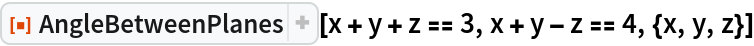Out=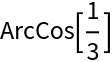### Scope (2)

Find the angle between two planes:

 In:=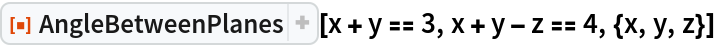Out=Find the angle between two planes:

 In:=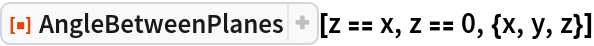Out=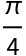Plot the two planes:

 In:=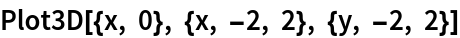Out=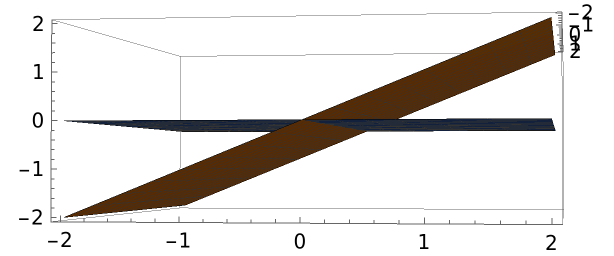Obtain a half plane representation of the angle:

 In:=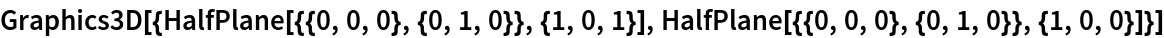Out=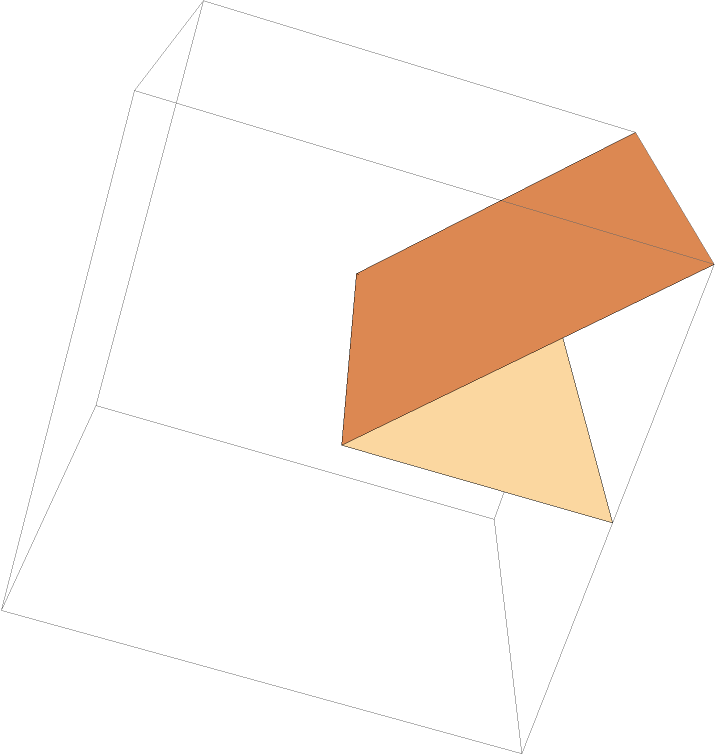Verify the angles are equal:

 In:=Out=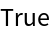### Possible Issues (1)

The functions must be linear:

 In:=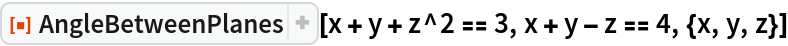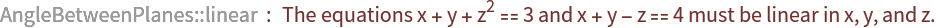Out=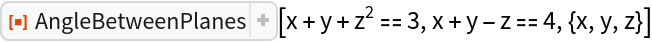## Publisher

Wolfram|Alpha Math Team

## Version History

• 2.0.0 – 23 March 2023
• 1.0.0 – 07 July 2021

## Author Notes

To view the full source code for AngleBetweenPlanes, evaluate the following:

 In:=